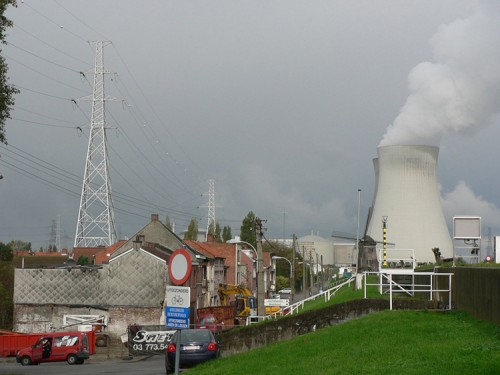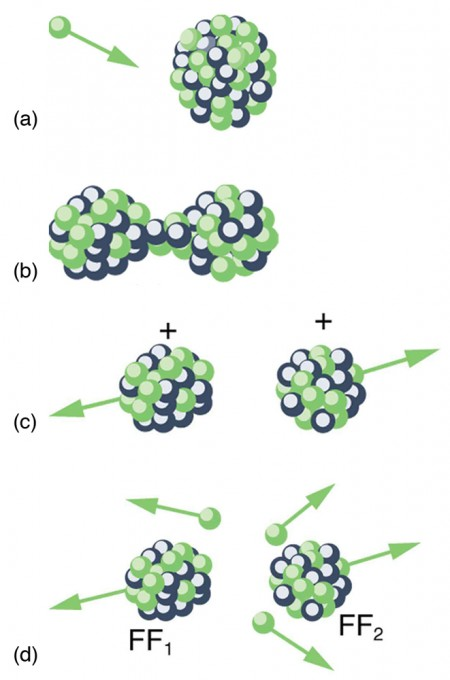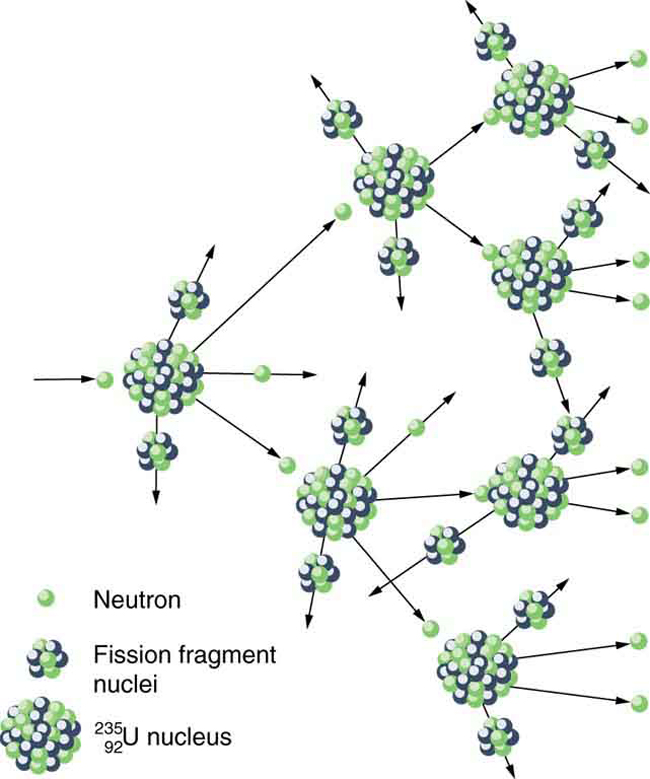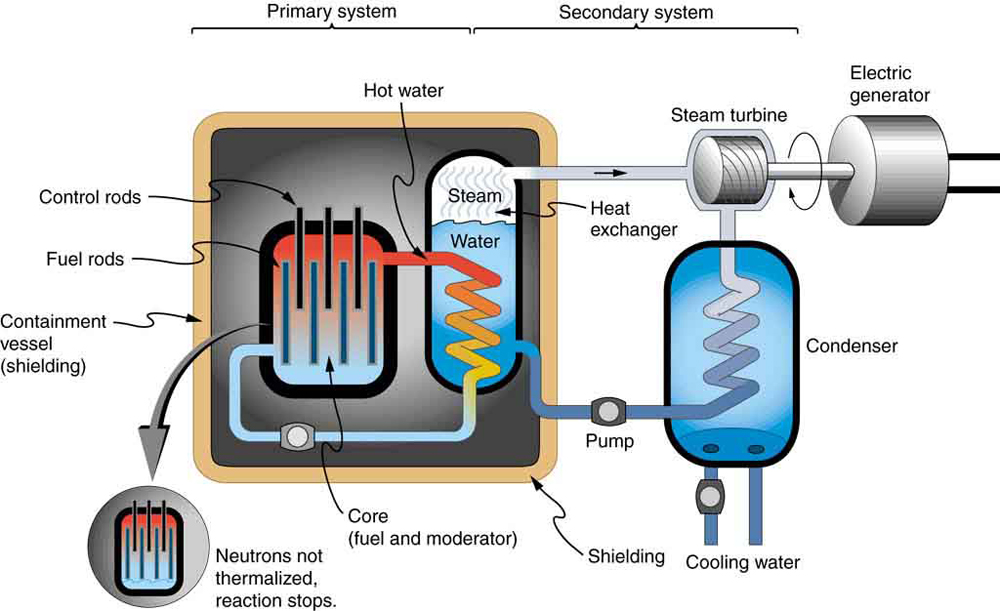## Fission

### LEARNING OBJECTIVES

By the end of this section, you will be able to:

• Define nuclear fission.
• Discuss how fission fuel reacts and describe what it produces.
• Describe controlled and uncontrolled chain reactions.

Nuclear fission is a reaction in which a nucleus is split (or fissured). Controlled fission is a reality, whereas controlled fusion is a hope for the future. Hundreds of nuclear fission power plants around the world attest to the fact that controlled fission is practical and, at least in the short term, economical, as seen in Figure 1. Whereas nuclear power was of little interest for decades following TMI and Chernobyl (and now Fukushima Daiichi), growing concerns over global warming has brought nuclear power back on the table as a viable energy alternative. By the end of 2009, there were 442 reactors operating in 30 countries, providing 15% of the world’s electricity. France provides over 75% of its electricity with nuclear power, while the US has 104 operating reactors providing 20% of its electricity. Australia and New Zealand have none. China is building nuclear power plants at the rate of one start every month.Figure 1. The people living near this nuclear power plant have no measurable exposure to radiation that is traceable to the plant. About 16% of the world’s electrical power is generated by controlled nuclear fission in such plants. The cooling towers are the most prominent features but are not unique to nuclear power. The reactor is in the small domed building to the left of the towers. (credit: Kalmthouts)

Fission is the opposite of fusion and releases energy only when heavy nuclei are split. As noted in Fusion, energy is released if the products of a nuclear reaction have a greater binding energy per nucleon (BE/A) than the parent nuclei. Figure 2 shows that BE/A is greater for medium-mass nuclei than heavy nuclei, implying that when a heavy nucleus is split, the products have less mass per nucleon, so that mass is destroyed and energy is released in the reaction. The amount of energy per fission reaction can be large, even by nuclear standards. The graph in Figure 2 shows BE/A to be about 7.6 MeV/nucleon for the heaviest nuclei (A about 240), while BE/A is about 8.6 MeV/nucleon for nuclei having A about 120. Thus, if a heavy nucleus splits in half, then about 1 MeV per nucleon, or approximately 240 MeV per fission, is released. This is about 10 times the energy per fusion reaction, and about 100 times the energy of the average α, β, or γ decay.

### Example 1. Calculating Energy Released by Fission

Calculate the energy released in the following spontaneous fission reaction:

238U → 95Sr + 140Xe + 3n

given the atomic masses to be m(238U) = 238.050784 u, m(95Sr) = 94.919388 u, m(140Xe) = 139.921610 u, and m(n) =1.008665 u.

#### Strategy

As always, the energy released is equal to the mass destroyed times c2, so we must find the difference in mass between the parent 238U and the fission products.

#### Solution

The products have a total mass of

$\begin{array}{lll}{m}_{\text{products}}& =& 94.919388\text{ u}+139.921610 \text{ u}+3\left(1.008665\text{ u}\right)\\ & =& 237.866993\text{ u}\end{array}\\$

The mass lost is the mass of 238U minus mproducts, or

Δ= 238.050784 u− 237.8669933 u = 0.183791 u,

so the energy released is

$\begin{array}{lll}E& =& \left(\Delta m\right){c}^{2}\\ & =& \left(0.183791\text{ u}\right)\frac{931.5\text{ Me}\text{V/}{c}^{2}}{\text{u}}{c}^{2}=171.2\text{ MeV}\end{array}\\$

#### Discussion

A number of important things arise in this example. The 171-MeV energy released is large, but a little less than the earlier estimated 240 MeV. This is because this fission reaction produces neutrons and does not split the nucleus into two equal parts. Fission of a given nuclide, such as 238U , does not always produce the same products. Fission is a statistical process in which an entire range of products are produced with various probabilities. Most fission produces neutrons, although the number varies with each fission. This is an extremely important aspect of fission, because neutrons can induce more fission, enabling self-sustaining chain reactions.

Spontaneous fission can occur, but this is usually not the most common decay mode for a given nuclide. For example, 238U can spontaneously fission, but it decays mostly by α emission. Neutron-induced fission is crucial as seen in Figure 2. Being chargeless, even low-energy neutrons can strike a nucleus and be absorbed once they feel the attractive nuclear force. Large nuclei are described by a liquid drop model with surface tension and oscillation modes, because the large number of nucleons act like atoms in a drop. The neutron is attracted and thus, deposits energy, causing the nucleus to deform as a liquid drop. If stretched enough, the nucleus narrows in the middle. The number of nucleons in contact and the strength of the nuclear force binding the nucleus together are reduced. Coulomb repulsion between the two ends then succeeds in fissioning the nucleus, which pops like a water drop into two large pieces and a few neutrons. Neutron-induced fission can be written as

A→ FFFFxn,

where FF1 and FF2 are the two daughter nuclei, called fission fragments, and x is the number of neutrons produced. Most often, the masses of the fission fragments are not the same. Most of the released energy goes into the kinetic energy of the fission fragments, with the remainder going into the neutrons and excited states of the fragments. Since neutrons can induce fission, a self-sustaining chain reaction is possible, provided more than one neutron is produced on average — that is, if x>1 in A→ FFFFxn. This can also be seen in Figure 3. An example of a typical neutron-induced fission reaction is

$n+{}_{\text{92}}^{\text{235}}\text{U}\to {}_{\text{56}}^{\text{142}}\text{Ba}+{}_{\text{36}}^{\text{91}}\text{Kr}+3\text{n}\\$.

Note that in this equation, the total charge remains the same (is conserved): 92 + 0 = 56 + 36. Also, as far as whole numbers are concerned, the mass is constant: 1 + 235 = 142 + 91 + 3. This is not true when we consider the masses out to 6 or 7 significant places, as in the previous example.Figure 2. Neutron-induced fission is shown. First, energy is put into this large nucleus when it absorbs a neutron. Acting like a struck liquid drop, the nucleus deforms and begins to narrow in the middle. Since fewer nucleons are in contact, the repulsive Coulomb force is able to break the nucleus into two parts with some neutrons also flying away.Figure 3. A chain reaction can produce self-sustained fission if each fission produces enough neutrons to induce at least one more fission. This depends on several factors, including how many neutrons are produced in an average fission and how easy it is to make a particular type of nuclide fission.

Not every neutron produced by fission induces fission. Some neutrons escape the fissionable material, while others interact with a nucleus without making it fission. We can enhance the number of fissions produced by neutrons by having a large amount of fissionable material. The minimum amount necessary for self-sustained fission of a given nuclide is called its critical mass. Some nuclides, such as 239Pu, produce more neutrons per fission than others, such as 235U . Additionally, some nuclides are easier to make fission than others. In particular, 235U and 239Pu, are easier to fission than the much more abundant 238U . Both factors affect critical mass, which is smallest for 239Pu.

The reason 235U and 239Pu are easier to fission than 238U is that the nuclear force is more attractive for an even number of neutrons in a nucleus than for an odd number. Consider that ${}_{\text{92}}^{\text{235}}{\text{U}}_{\text{143}}\\$ has 143 neutrons, and ${}_{\text{94}}^{\text{239}}{\text{P}}_{\text{145}}\\$ has 145 neutrons, whereas ${}_{\text{92}}^{\text{238}}{\text{U}}_{\text{146}}\\$ has 146. When a neutron encounters a nucleus with an odd number of neutrons, the nuclear force is more attractive, because the additional neutron will make the number even. About 2-MeV more energy is deposited in the resulting nucleus than would be the case if the number of neutrons was already even. This extra energy produces greater deformation, making fission more likely. Thus, 235U and 239Pu are superior fission fuels. The isotope 235U is only 0.72 % of natural uranium, while 238U is 99.27%, and 239Pu does not exist in nature. Australia has the largest deposits of uranium in the world, standing at 28% of the total. This is followed by Kazakhstan and Canada. The US has only 3% of global reserves.

Most fission reactors utilize 235U , which is separated from 238U at some expense. This is called enrichment. The most common separation method is gaseous diffusion of uranium hexafluoride (UF6) through membranes. Since 235U has less mass than 238U , its UF6 molecules have higher average velocity at the same temperature and diffuse faster. Another interesting characteristic of 235U is that it preferentially absorbs very slow moving neutrons (with energies a fraction of an eV), whereas fission reactions produce fast neutrons with energies in the order of an MeV. To make a self-sustained fission reactor with 235U , it is thus necessary to slow down (“thermalize”) the neutrons. Water is very effective, since neutrons collide with protons in water molecules and lose energy. Figure 4 shows a schematic of a reactor design, called the pressurized water reactor.Figure 4. A pressurized water reactor is cleverly designed to control the fission of large amounts of 235U , while using the heat produced in the fission reaction to create steam for generating electrical energy. Control rods adjust neutron flux so that criticality is obtained, but not exceeded. In case the reactor overheats and boils the water away, the chain reaction terminates, because water is needed to thermalize the neutrons. This inherent safety feature can be overwhelmed in extreme circumstances.

Control rods containing nuclides that very strongly absorb neutrons are used to adjust neutron flux. To produce large power, reactors contain hundreds to thousands of critical masses, and the chain reaction easily becomes self-sustaining, a condition called criticality. Neutron flux should be carefully regulated to avoid an exponential increase in fissions, a condition called supercriticality. Control rods help prevent overheating, perhaps even a meltdown or explosive disassembly. The water that is used to thermalize neutrons, necessary to get them to induce fission in 235U, and achieve criticality, provides a negative feedback for temperature increases. In case the reactor overheats and boils the water to steam or is breached, the absence of water kills the chain reaction. Considerable heat, however, can still be generated by the reactor’s radioactive fission products. Other safety features, thus, need to be incorporated in the event of a loss of coolant accident, including auxiliary cooling water and pumps.

### Example 2. Calculating Energy from a Kilogram of Fissionable Fuel

Calculate the amount of energy produced by the fission of 1.00 kg of 235U , given the average fission reaction of 235U produces 200 MeV.

#### Strategy

The total energy produced is the number of 235U atoms times the given energy per 235 U fission. We should therefore find the number of 235U atoms in 1.00 kg.

#### Solution

The number of 235U atoms in 1.00 kg is Avogadro’s number times the number of moles. One mole of 235U has a mass of 235.04 g; thus, there are (1000 g)/(235.04 g/mol) = 4.25 mol. The number of 235U atoms is therefore,

$\left(4.25 \text{ mol}\right)\left(6.02\times {10}^{23}{}^{\{235}\text{U/mol}\right)=2.56\times{10}^{24}{}^\text{ 235}\text{U}\\$.

So the total energy released is

$\begin{array}{lll}E & =& \left(2.56\times {10}^{24}{}^{235}\text{U}\right)\left(\frac{200\text{ MeV}}{{}^{\text{235}}\text{U}}\right)\left(\frac{1.60\times {10}^{-13}\text{ J}}{\text{MeV}}\right)\\ & =& 8.21\times {10}^{13}\text{ J}\end{array}\\$.

#### Discussion

This is another impressively large amount of energy, equivalent to about 14,000 barrels of crude oil or 600,000 gallons of gasoline. But, it is only one-fourth the energy produced by the fusion of a kilogram mixture of deuterium and tritium as seen in Example 1. Calculating Energy and Power from Fusion. Even though each fission reaction yields about ten times the energy of a fusion reaction, the energy per kilogram of fission fuel is less, because there are far fewer moles per kilogram of the heavy nuclides. Fission fuel is also much more scarce than fusion fuel, and less than 1% of uranium (the 235U) is readily usable.

One nuclide already mentioned is 239Pu, which has a 24,120-y half-life and does not exist in nature. Plutonium-239 is manufactured from 238U in reactors, and it provides an opportunity to utilize the other 99% of natural uranium as an energy source. The following reaction sequence, called breeding, produces 239Pu. Breeding begins with neutron capture by 238U :

238U + → 239U + γ.

Uranium-239 then β decays:

239 239Np β− + ve(t1/2 23 min).

Neptunium-239 also β decays:

239Np → 239Pu β− ve(t1/2 2.d).

Plutonium-239 builds up in reactor fuel at a rate that depends on the probability of neutron capture by 238U (all reactor fuel contains more 238U than 235U). Reactors designed specifically to make plutonium are called breeder reactors. They seem to be inherently more hazardous than conventional reactors, but it remains unknown whether their hazards can be made economically acceptable. The four reactors at Chernobyl, including the one that was destroyed, were built to breed plutonium and produce electricity. These reactors had a design that was significantly different from the pressurized water reactor illustrated above. Plutonium-239 has advantages over 235U as a reactor fuel — it produces more neutrons per fission on average, and it is easier for a thermal neutron to cause it to fission. It is also chemically different from uranium, so it is inherently easier to separate from uranium ore. This means 239Pu has a particularly small critical mass, an advantage for nuclear weapons.

## PhET Explorations: Nuclear Fission

Start a chain reaction, or introduce non-radioactive isotopes to prevent one. Control energy production in a nuclear reactor!

## Section Summary

• Nuclear fission is a reaction in which a nucleus is split.
• Fission releases energy when heavy nuclei are split into medium-mass nuclei.
• Self-sustained fission is possible, because neutron-induced fission also produces neutrons that can induce other fissions, A→ FF+ FFxn, where FF1 and FF2 are the two daughter nuclei, or fission fragments, and x is the number of neutrons produced.
• A minimum mass, called the critical mass, should be present to achieve criticality.
• More than a critical mass can produce supercriticality.
• The production of new or different isotopes (especially 239Pu) by nuclear transformation is called breeding, and reactors designed for this purpose are called breeder reactors.

### Conceptual Questions

1. Explain why the fission of heavy nuclei releases energy. Similarly, why is it that energy input is required to fission light nuclei?
2. Explain, in terms of conservation of momentum and energy, why collisions of neutrons with protons will thermalize neutrons better than collisions with oxygen.
3. The ruins of the Chernobyl reactor are enclosed in a huge concrete structure built around it after the accident. Some rain penetrates the building in winter, and radioactivity from the building increases. What does this imply is happening inside?
4. Since the uranium or plutonium nucleus fissions into several fission fragments whose mass distribution covers a wide range of pieces, would you expect more residual radioactivity from fission than fusion? Explain.
5. The core of a nuclear reactor generates a large amount of thermal energy from the decay of fission products, even when the power-producing fission chain reaction is turned off. Would this residual heat be greatest after the reactor has run for a long time or short time? What if the reactor has been shut down for months?
6. How can a nuclear reactor contain many critical masses and not go supercritical? What methods are used to control the fission in the reactor?
7. Why can heavy nuclei with odd numbers of neutrons be induced to fission with thermal neutrons, whereas those with even numbers of neutrons require more energy input to induce fission?
8. Why is a conventional fission nuclear reactor not able to explode as a bomb?

### Problems & Exercises

1. (a) Calculate the energy released in the neutron-induced fission (similar to the spontaneous fission in Example 1. Calculating Energy Released by Fission)

238U → 96Sr + 140Xe + 3n,

given m(96Sr) = 95.921750 u and m(140Xe) = 139.92164. (b) This result is about 6 MeV greater than the result for spontaneous fission. Why? (c) Confirm that the total number of nucleons and total charge are conserved in this reaction.

2. (a) Calculate the energy released in the neutron-induced fission reaction

n + 235U → 92Kr + 142Ba + 2n,

given m(92Kr) = 91.926269 u and m(142Ba) = 141.916361 u.

(b) Confirm that the total number of nucleons and total charge are conserved in this reaction.

3. (a) Calculate the energy released in the neutron-induced fission reaction

239Pu → 96Sr + 140Ba + 4n,
given m(96Sr) = 95.921750 u and m(140Ba) = 139.910581 u.

(b) Confirm that the total number of nucleons and total charge are conserved in this reaction.

4. Confirm that each of the reactions listed for plutonium breeding just following Example 2. Calculating Energy from a Kilogram of Fissionable Fuel conserves the total number of nucleons, the total charge, and electron family number.

5. Breeding plutonium produces energy even before any plutonium is fissioned. (The primary purpose of the four nuclear reactors at Chernobyl was breeding plutonium for weapons. Electrical power was a by-product used by the civilian population.) Calculate the energy produced in each of the reactions listed for plutonium breeding just following Example 2. Calculating Energy from a Kilogram of Fissionable Fuel. The pertinent masses are m(239U) = 239.054289 u, m(239Np) = 239.052932 u, and m(239Pu) = 239.052157 u.

6. The naturally occurring radioactive isotope 232Th does not make good fission fuel, because it has an even number of neutrons; however, it can be bred into a suitable fuel (much as 238U is bred into 239P).

(a) What are Z and N for 232Th?

(b) Write the reaction equation for neutron captured by 232Th and identify the nuclide AX produced in 232Th → Aγ.

(c) The product nucleus β decays, as does its daughter. Write the decay equations for each, and identify the final nucleus.

(d) Confirm that the final nucleus has an odd number of neutrons, making it a better fission fuel.

(e) Look up the half-life of the final nucleus to see if it lives long enough to be a useful fuel.

7. The electrical power output of a large nuclear reactor facility is 900 MW. It has a 35.0% efficiency in converting nuclear power to electrical.

(a) What is the thermal nuclear power output in megawatts?

(b) How many 235U nuclei fission each second, assuming the average fission produces 200 MeV?

(c) What mass of 235U is fissioned in one year of full-power operation?

8. A large power reactor that has been in operation for some months is turned off, but residual activity in the core still produces 150 MW of power. If the average energy per decay of the fission products is 1.00 MeV, what is the core activity in curies?

## Glossary

breeder reactors:
reactors that are designed specifically to make plutonium
breeding:
reaction process that produces 239Pu
criticality:
condition in which a chain reaction easily becomes self-sustaining
critical mass:
minimum amount necessary for self-sustained fission of a given nuclide
fission fragments:
a daughter nuclei
liquid drop model:
a model of nucleus (only to understand some of its features) in which nucleons in a nucleus act like atoms in a drop
nuclear fission:
reaction in which a nucleus splits
neutron-induced fission:
fission that is initiated after the absorption of neutron
supercriticality:
an exponential increase in fissions

### Selected Solutions to Problems & Exercises

1. (a) 177.1 MeV (b) Because the gain of an external neutron yields about 6 MeV, which is the average BE/A for heavy nuclei. (c) A = 1 + 238 = 96 + 140 + 1 + 1 + 1, Z = 92 = 38 + 53, efn = 0 = 0

3. (a) 180.6 MeV (b)     A = 1 + 239 = 96 + 140 + 1 + 1 + 1 + 1, Z = 94 = 38 + 56, efn = 0 = 0

5. 238U + → 239U + γ 4.81 MeV

239U → 239Np + β− ve 0.753 MeV

239Np → 239Pu + β− ve 0.211 MeV

7. (a) 2.57 × 10MW (b) 8.03 × 1019 fission/s (c) 991 kg